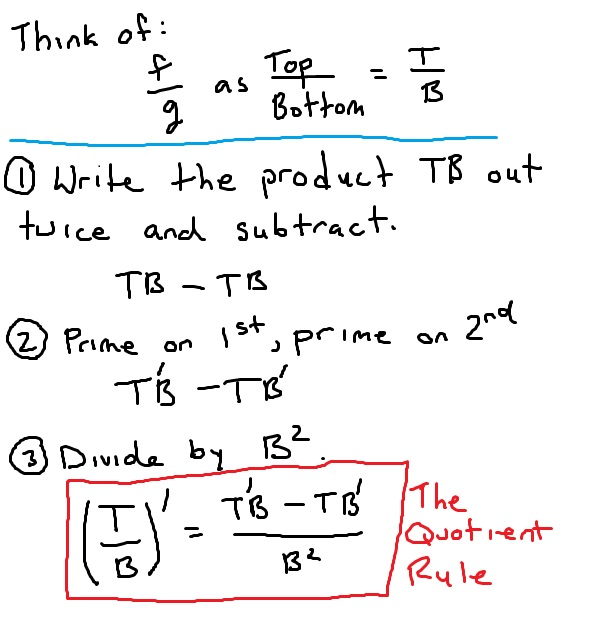﻿ The quotient rule - explanation and examples - MathBootCamps

# The quotient rule

There are many so-called “shortcut” rules for finding the derivative of a function. The quotient rule, is a rule used to find the derivative of a function that can be written as the quotient of two functions. More simply, you can think of the quotient rule as applying to functions that are written out as fractions, where the numerator and the denominator are both themselves functions.

## The formula for the quotient rule

For functions f and g, and using primes for the derivatives, the formula is:## Remembering the quotient rule

You can certainly just memorize the quotient rule and be set for finding derivatives, but you may find it easier to remember the pattern. This is shown below.## Examples

Naturally, the best way to understand how to use the quotient rule is to look at some examples. Notice that in each example below, the calculus step is much quicker than the algebra that follows. This is true for most questions where you apply the quotient rule. You will often need to simplify quite a bit to get the final answer.

### Example

Find the derivative of the function:
$$f(x) = \dfrac{x-1}{x+2}$$

### Solution

This is a fraction involving two functions, and so we first apply the quotient rule.

$$f^{\prime}(x) = \dfrac{(x-1)^{\prime}(x+2)-(x-1)(x+2)^{\prime}}{(x+2)^2}$$

Find the derivative for each prime.

$$f^{\prime}(x) = \dfrac{(1)(x+2)-(x-1)(1)}{(x+2)^2}$$

Simplify, if possible.

\begin{align}f^{\prime}(x) &= \dfrac{(x+2)-(x-1)}{(x+2)^2}\\ &= \dfrac{x+2-x+1}{(x+2)^2}\\ &= \boxed{\dfrac{3}{(x+2)^2}}\end{align}

When applying this rule, it may be that you work with more complicated functions than you just saw. In the next example, you will need to remember that:

$$(\ln x)^{\prime} = \dfrac{1}{x}$$

To review this rule, see: The derivative of the natural log

### Example

Find the derivative of the function:
$$y = \dfrac{\ln x}{2x^2}$$

### Solution

As above, this is a fraction involving two functions, so:
Apply the quotient rule.

$$y^{\prime} = \dfrac{(\ln x)^{\prime}(2x^2) – (\ln x)(2x^2)^{\prime}}{(2x^2)^2}$$

Find the derivative for each prime.

$$y^{\prime} = \dfrac{(\dfrac{1}{x})(2x^2) – (\ln x)(4x)}{(2x^2)^2}$$

Simplify, if possible.

\begin{align}y^{\prime} &= \dfrac{2x – 4x\ln x}{4x^4}\\ &= \dfrac{(2x)(1 – 2\ln x)}{4x^4}\\ &= \boxed{\dfrac{1 – 2\ln x}{2x^3}}\end{align}

## Hint: Save work by simplifying first

Once you have the hang of working with this rule, you may be tempted to apply it to any function written as a fraction, without thinking about possible simplification first. This could make you do much more work than you need to! Consider the following example.

### Example

Find the derivative of the function:
$$g(x) = \dfrac{1-x^2}{5x^2}$$

Given the form of this function, you could certainly apply the quotient rule to find the derivative. However, we can apply a little algebra first. Since the denominator is a single value, we can write:

$$g(x) = \dfrac{1-x^2}{5x^2} = \dfrac{1}{5x^2} – \dfrac{x^2}{5x^2} = \dfrac{1}{5x^2} – \dfrac{1}{5}$$

Now, using the definition of a negative exponent:

$$g(x) = \dfrac{1}{5x^2} – \dfrac{1}{5} = \dfrac{1}{5}x^{-2} – \dfrac{1}{5}$$

Now we can apply the power rule instead of the quotient rule:

\begin{align}g^{\prime}(x) &= \left(\dfrac{1}{5}x^{-2} – \dfrac{1}{5}\right)^{\prime}\\ &= \dfrac{-2}{5}x^{-3}\\ &= \boxed{\dfrac{-2}{5x^3}}\end{align}

In the example above, remember that the derivative of a constant is zero. This is why we no longer have $$\dfrac{1}{5}$$ in the answer.

Not bad right? For practice, you should try applying the quotient rule and verifying that you get the same answer.

## Continue your study of derivatives

Previous: The product rule
Next: The chain rule